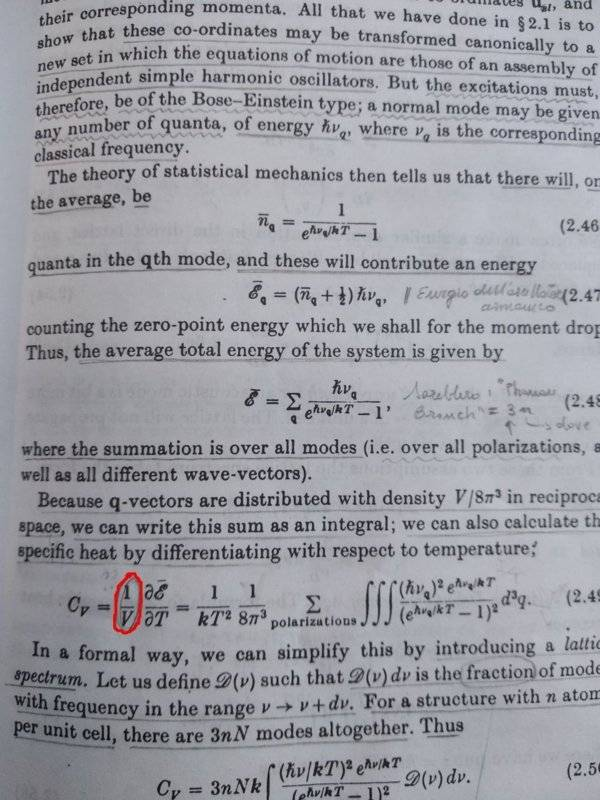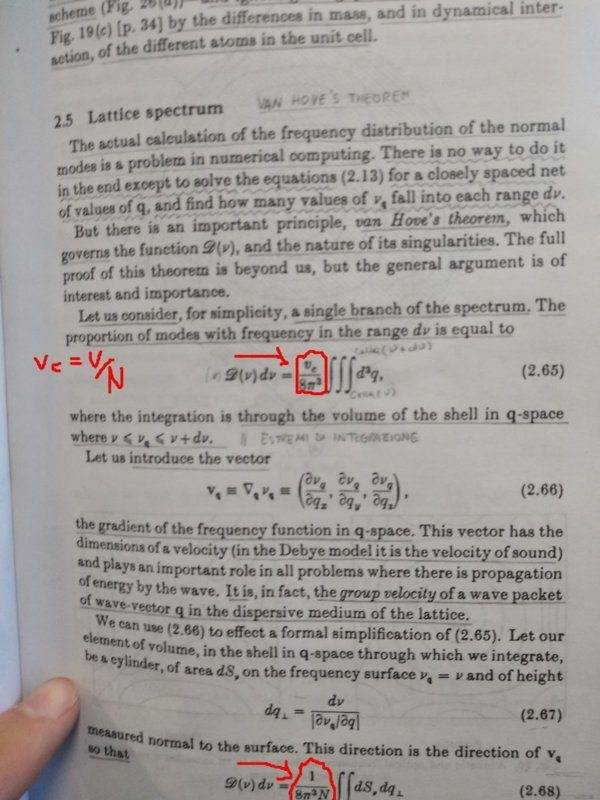# Lattice specific heat, help understanding a passage in Ziman's book

• I
• dRic2

#### dRic2

Gold Member
Hi, I don't understand why the author in calculating the expression for the specific heat, divides by ##1/V## (the total volume).Also, in calculating the fraction of modes with frequency in the range ##v##, ##v+dv## he divides by ##V## (eq. 2.65, 2.67)Thanks,
Ric

Energy is extensive (varies with system size), and dividing by another extensive quantity (volume) gives an intensive quantity. In this case, it’s volumetric heat capacity. Mass or molar heat capacity (where you divide the derivative of heat wrt temperature by mass or amount of substance) are more familiar, but it’s the same idea: you’re looking for a property which is independent of the size of the system.

Last edited:
•dRic2
Also, in calculating the fraction of modes with frequency in the range v, v+dv he divides by V (eq. 2.65, 2.67)

The same as indicated by @TeethWhitener: The phonon density of states gives the number of modes per unit frequency per unit volume of real space.

•dRic2
Thank you very much. I had to be sure because it was not explicitly specified, although it might be intuitive.